# How to Separate Numbers and Texts in Excel

When working in Microsoft Excel, you may encounter a situation where a cell's text is all jumbled up. Such a cell will contain numbers and texts, making it hard to decipher what it means. It mostly happens when importing data from other systems that your Excel program cannot read. Such a scenario can be so frustrating and time-consuming. To solve this scenario, it is advisable to separate the texts and numbers for your worksheet to make sense and be easily understandable. Excel has a lot of features that help make this possible.

In the article below, we will show you different methods you can take when you want to separate numbers and texts in Excel. Let's get started.

## Method 1: Using the Flash Fill feature to separate texts and numbers in Excel

1. In your open Excel workbook, select the cell that contains the numbers and texts you want to separate.2. In the adjacent blank cell, type in the characters of your first text string.3. Select all the cell ranges where you want to fill the numbers, and click the Data tab on the main menu ribbon.4. Select the Flash Fill option, and only the numbers will be filled in these cells.All the text will be separated5. In another column, one adjacent to the separated number column, type in the first number of the string6. Select all the cells below the first entry, and click Data> Flash Fill. Doing so will separate the numbers from the string.## Method 2: separating numbers and texts using formulas

You can easily separate your numbers from texts using Excel formulas. It may require the use of complex formulas, but the method is reliable. When it comes to separating texts, we use RIGHT, LEFT, MID, and other text functions. For this to be successful, a user has to know the exact number and texts to extract

The formula to use is =MIN(FIND({0, 1, 2, 3, 4, 5, 6, 7, 8, 9}, a2& "0123456789"))

So long as you understand the FIND function, the formula is pretty much easy to grasp. The function will run ten times, once for each number, and the MIN function will return the first number in the cell. Let's use the following data as an example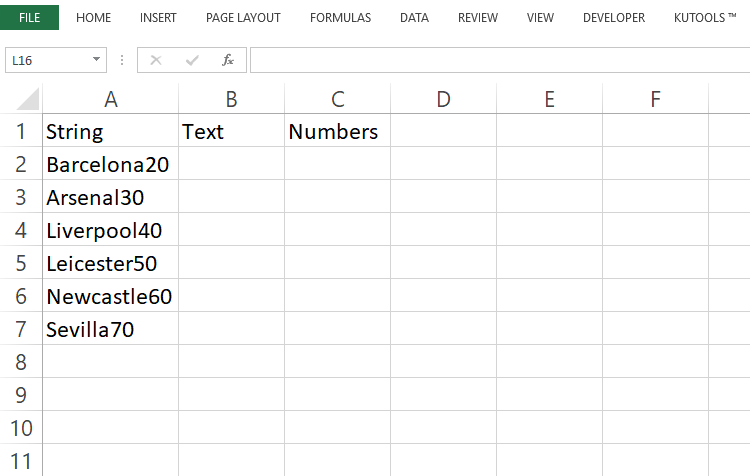1. To extract the names, enter this formula into a blank cell where you want to place the results =LEFT(A2,MIN(FIND({0, 1, 2, 3, 4, 5, 6, 7, 8, 9},A2& "0123456789"))-1)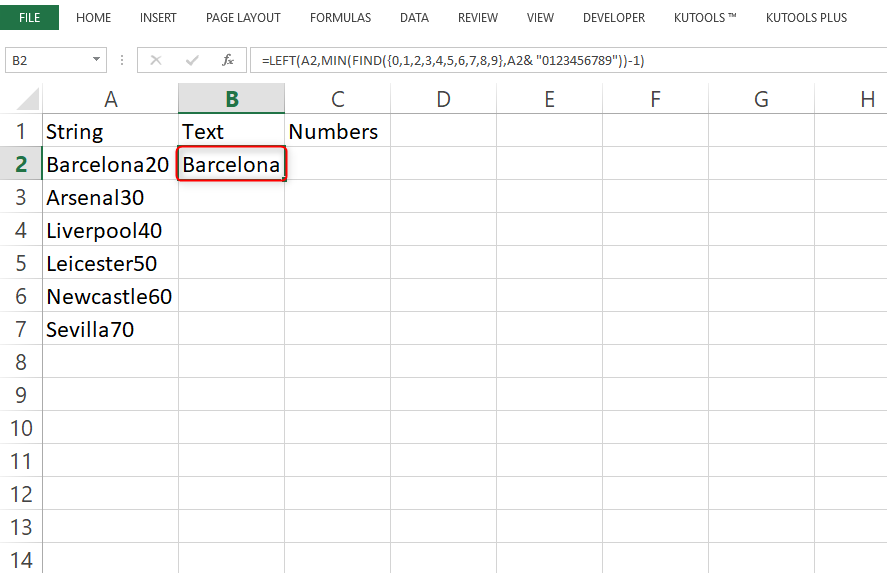2. To extract the numbers, enter this formula into a black cell that will hold the results =RIGHT(A2,LEN(A2)-MIN(FIND({0, 1, 2, 3, 4, 5, 6, 7, 8, 9},A2& "0123456789"))+1) , (A2 IS THE CELL WHICH CONTAINS THE TEXT STRING YOU WANT TO SEPARATE)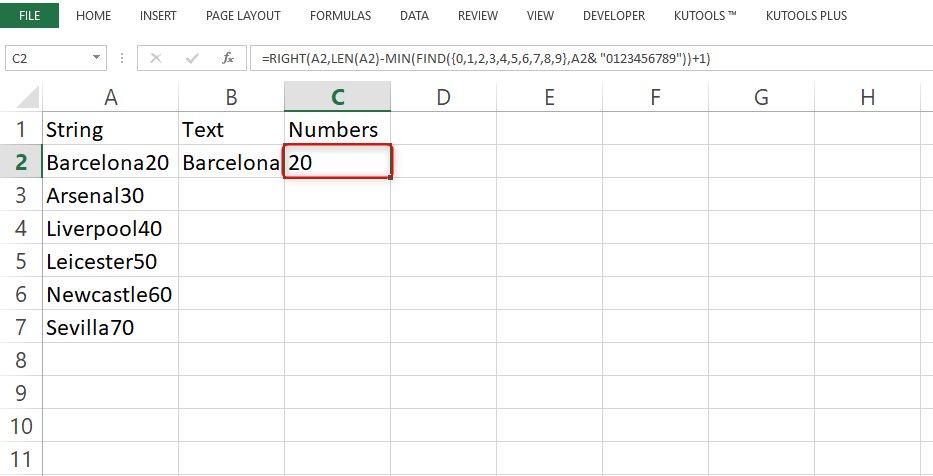3. After filling up these formulas press the Enter key, and the texts and numbers will separate.

4. To separate the other text and number strings, select the first two separated cells and drag the fill handle.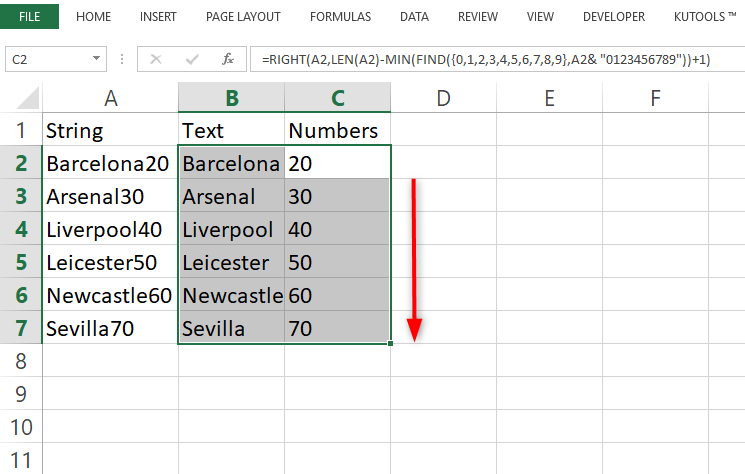Method 3: separating irregularly mixed texts and numbers using VBA code

1. Hold down the Alt + F11 keys to open the VBA editor window.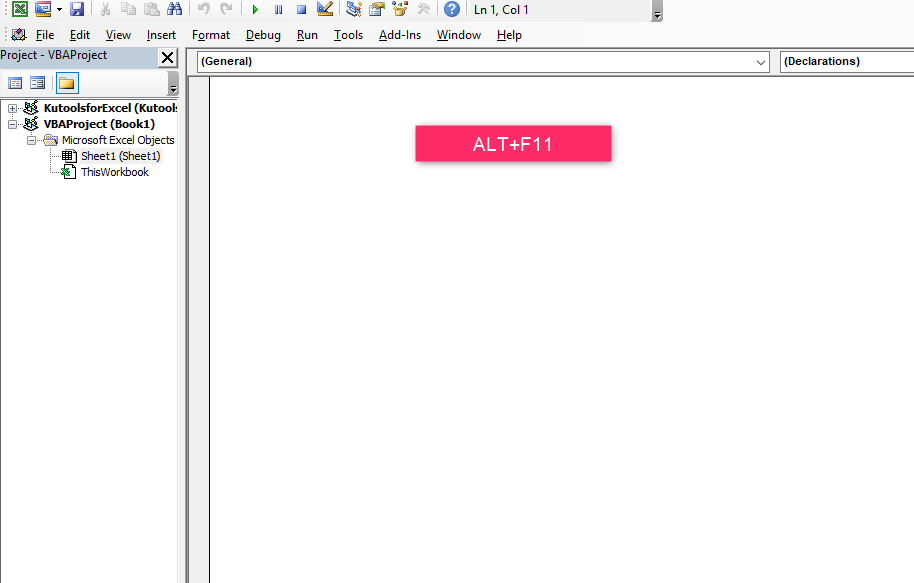2. Click insert and select Module. In the open Module window, paste or type in your code. For example, you can use the code below to separate texts and numbers into different cells from one cell.

```Option Explicit Public Function Strip(ByVal x As String, LeaveNums As Boolean) As Variant Dim y As String, z As String, n As Long For n = 1 To Len(x) y = Mid(x, n, 1) If LeaveNums = False Then If y Like "[A-Za-z ]" Then z = z & y 'False keeps Letters and spaces only Else If y Like "[0-9. ]" Then z = z & y 'True keeps Numbers and decimal points End If Next n Strip = Trim(z) End Function ```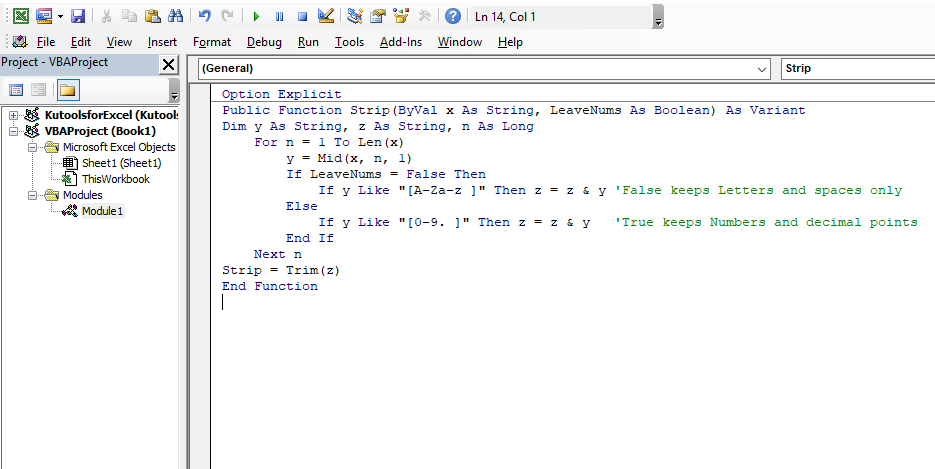3. Save and close the code window to return to your worksheet. In a blank cell, enter the formula =Strip(A2,FALSE) to extract the text string only. Drag the fill handle to the cells you want to fill.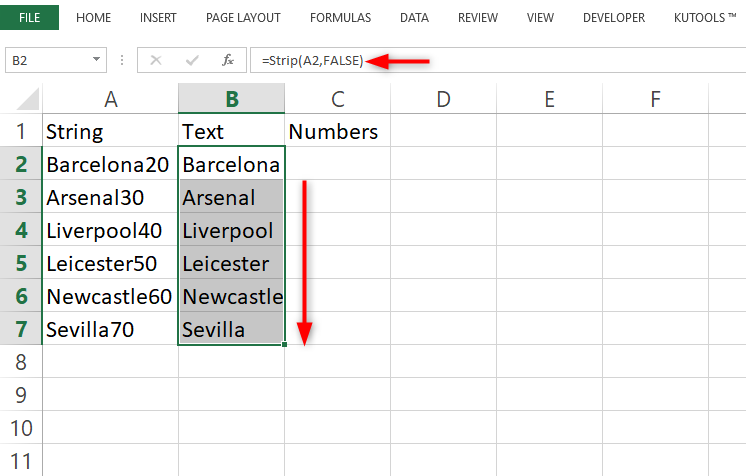4. In another blank cell, type in the formula =NUMBERVALUE(Strip(A2,TRUE)) . Drag the fill handle downwards to other cells to fill in only the numbers.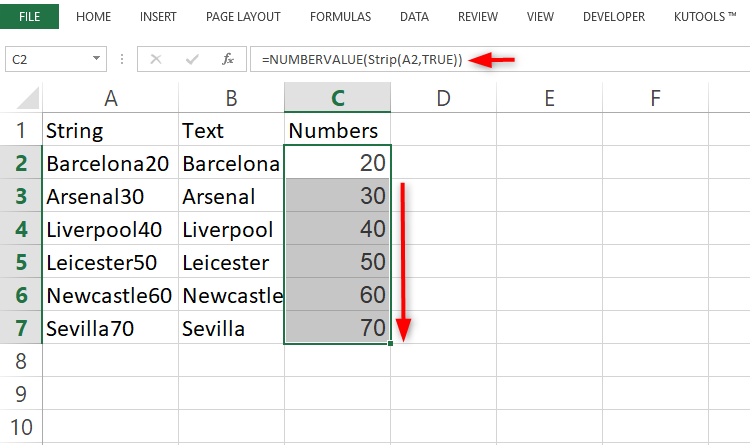Note that, while using this method, the result may be incorrect when there are any decimal numbers in your text string.